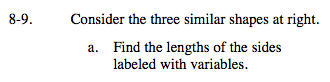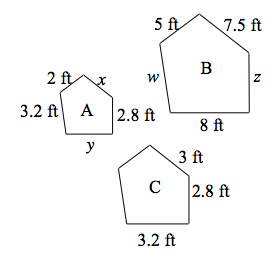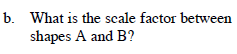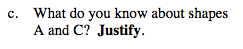### Home > MC2 > Chapter 8 > Lesson 8.1.1 > Problem8-9

8-9.Find the scale factor between shapes A and B. To do so, compare two matching sides (one from each shape)
and find the ratio of their lengths.

Use this scale factor to divide or multiply the respective sides and solve for the unknown sides.

x = 3 ft
z = 7 ftWhat is the scale factor you found from part (a)?Shapes A and C are congruent, because they have a scale factor of 1 (all side lengths and angles are the same).